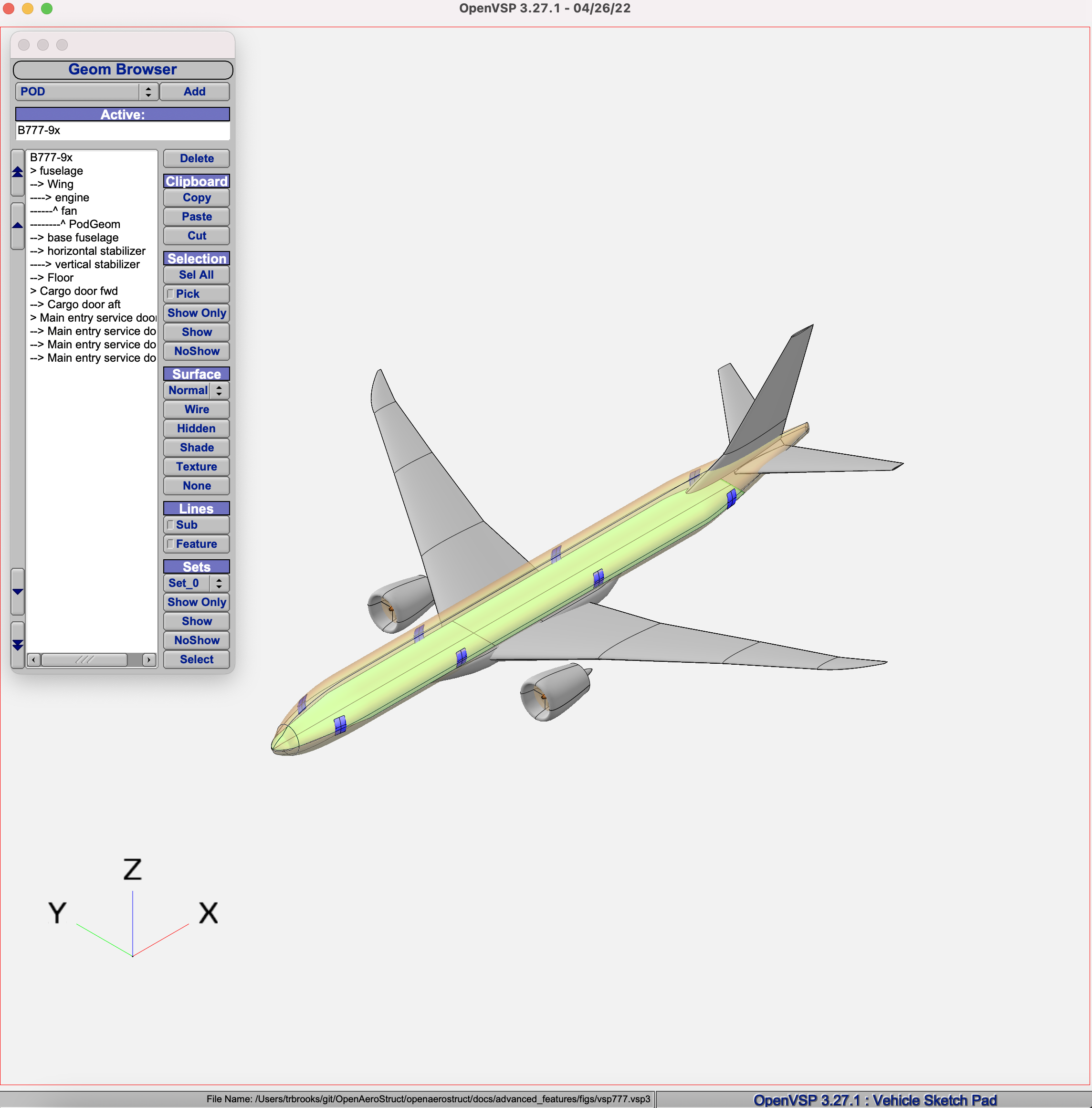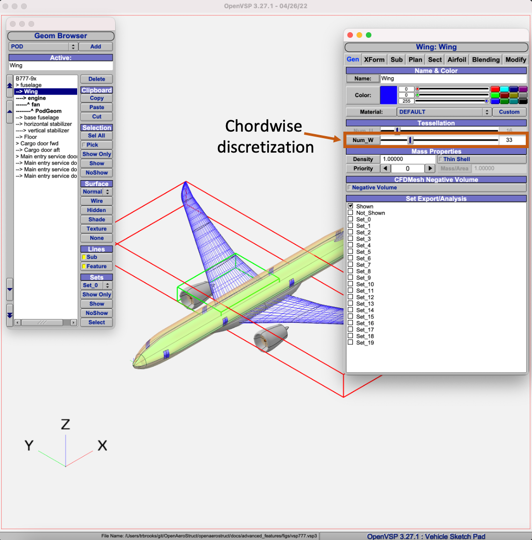# OpenVSP-generated Mesh Example

OpenAeroStruct also has the ability to generate VLM meshes from an OpenVSP `.vsp3` file. In order to use this feature, users must have the OpenVSP Python API installed in their Python environment. For instructions on how to install OpenVSP’s Python API see here.

Here is an example script with an aero surface mesh generated for a Boeing 777 aircraft defined in an OpenVSP model. This should help you understand how meshes are defined in OpenAeroStruct and how to create them for your own custom planform shapes. This is yet another alternative to the mesh definition approaches described in Aerodynamic Optimization and User-Provided Mesh Example.

The OpenVSP model used in this example is shown below:The following shows the portion of the example script in which the user provides the OpenVSP model for the mesh. In this example we’ll only be importing the wing, horizontal, and vertical tail from the OpenVSP model shown above.

```# ----------------------------------------------------------------------
# OPENVSP SURFACES: Example of OAS surfaces generated from OpenVSP model
# ----------------------------------------------------------------------
# VSP model
vsp_file = os.path.join(os.path.dirname(__file__), "Boeing_777-9x_ref.vsp3")

# Generate half-body mesh of 777, include only wing and tail surfaces
surfaces = generate_vsp_surfaces(
vsp_file, symmetry=True, include=["Wing", "horizontal stabilizer", "vertical stabilizer"]
)

# Define input surface dictionary for our wing
surf_options = {
"type": "aero",
"S_ref_type": "wetted",  # how we compute the wing area,
# can be 'wetted' or 'projected'
"twist_cp": np.zeros(3),  # Define twist using 3 B-spline cp's
# Aerodynamic performance of the lifting surface at
# an angle of attack of 0 (alpha=0).
# These CL0 and CD0 values are added to the CL and CD
# obtained from aerodynamic analysis of the surface to get
# the total CL and CD.
# These CL0 and CD0 values do not vary wrt alpha.
"CL0": 0.0,  # CL of the surface at alpha=0
"CD0": 0.0,  # CD of the surface at alpha=0
# Airfoil properties for viscous drag calculation
"k_lam": 0.05,  # percentage of chord with laminar
# flow, used for viscous drag
"t_over_c": 0.12,  # thickness over chord ratio (NACA0015)
"c_max_t": 0.303,  # chordwise location of maximum (NACA0015)
# thickness
"with_viscous": False,  # if true, compute viscous drag,
"with_wave": False,
}  # end of surface dictionary

# Update each surface with default options
for surface in surfaces:
surface.update(surf_options)

# -----------------------------------------------------------------------------
# END SURFACES
# -----------------------------------------------------------------------------
```

The following shows a visualization of the mesh.The complete script for the aerodynamic analysis is as follows.

```"""
Perform compressible inviscid aerodynamic anlysis on a Boeing 777-9X defined in an OpenVSP model.
Print out lift and drag coefficient when complete.

777 vsp model avaialable here: http://hangar.openvsp.org/vspfiles/375
"""
import os
import numpy as np

import openmdao.api as om

from openaerostruct.geometry.utils import generate_vsp_surfaces
from openaerostruct.geometry.geometry_group import Geometry
from openaerostruct.aerodynamics.aero_groups import AeroPoint

# docs checkpoint 0
# ----------------------------------------------------------------------
# OPENVSP SURFACES: Example of OAS surfaces generated from OpenVSP model
# ----------------------------------------------------------------------
# VSP model
vsp_file = os.path.join(os.path.dirname(__file__), "Boeing_777-9x_ref.vsp3")

# Generate half-body mesh of 777, include only wing and tail surfaces
surfaces = generate_vsp_surfaces(
vsp_file, symmetry=True, include=["Wing", "horizontal stabilizer", "vertical stabilizer"]
)

# Define input surface dictionary for our wing
surf_options = {
"type": "aero",
"S_ref_type": "wetted",  # how we compute the wing area,
# can be 'wetted' or 'projected'
"twist_cp": np.zeros(3),  # Define twist using 3 B-spline cp's
# Aerodynamic performance of the lifting surface at
# an angle of attack of 0 (alpha=0).
# These CL0 and CD0 values are added to the CL and CD
# obtained from aerodynamic analysis of the surface to get
# the total CL and CD.
# These CL0 and CD0 values do not vary wrt alpha.
"CL0": 0.0,  # CL of the surface at alpha=0
"CD0": 0.0,  # CD of the surface at alpha=0
# Airfoil properties for viscous drag calculation
"k_lam": 0.05,  # percentage of chord with laminar
# flow, used for viscous drag
"t_over_c": 0.12,  # thickness over chord ratio (NACA0015)
"c_max_t": 0.303,  # chordwise location of maximum (NACA0015)
# thickness
"with_viscous": False,  # if true, compute viscous drag,
"with_wave": False,
}  # end of surface dictionary

# Update each surface with default options
for surface in surfaces:
surface.update(surf_options)

# -----------------------------------------------------------------------------
# END SURFACES
# -----------------------------------------------------------------------------
# docs checkpoint 1

# Instantiate the problem and the model group
prob = om.Problem()

# Define flight variables as independent variables of the model
indep_var_comp = om.IndepVarComp()
indep_var_comp.add_output("v", val=248.136, units="m/s")  # Freestream Velocity
indep_var_comp.add_output("alpha", val=5.0, units="deg")  # Angle of Attack
indep_var_comp.add_output("beta", val=0.0, units="deg")  # Sideslip angle
indep_var_comp.add_output("omega", val=np.zeros(3), units="deg/s")  # Rotation rate
indep_var_comp.add_output("Mach_number", val=0.0)  # Freestream Mach number
indep_var_comp.add_output("re", val=1.0e6, units="1/m")  # Freestream Reynolds number
indep_var_comp.add_output("rho", val=0.38, units="kg/m**3")  # Freestream air density
indep_var_comp.add_output("cg", val=np.zeros((3)), units="m")  # Aircraft center of gravity
# Add vars to model, promoting is a quick way of automatically connecting inputs
# and outputs of different OpenMDAO components

# Add geometry group to the problem and add wing surface as a sub group.
# These groups are responsible for manipulating the geometry of the mesh,
# in this case spanwise twist.
geom_group = om.Group()
for surface in surfaces:

# Create the aero point group for this flight condition and add it to the model
aero_group = AeroPoint(surfaces=surfaces, rotational=True, compressible=True)
point_name = "flight_condition_0"
point_name, aero_group, promotes_inputs=["v", "alpha", "beta", "omega", "Mach_number", "re", "rho", "cg"]
)

# Connect the mesh from the geometry component to the analysis point
for surface in surfaces:
name = surface["name"]
prob.model.connect(name + ".mesh", point_name + "." + name + ".def_mesh")

# Perform the connections with the modified names within the
# 'aero_states' group.
prob.model.connect(name + ".mesh", point_name + ".aero_states." + name + "_def_mesh")

# Set optimizer as model driver (Just evaluate a single point)
prob.driver = om.ScipyOptimizeDriver(maxiter=1)
prob.driver.options["debug_print"] = ["nl_cons", "objs", "desvars"]

recorder = om.SqliteRecorder("vsp_777.db")
prob.driver.recording_options["record_derivatives"] = True
prob.driver.recording_options["includes"] = ["*"]

# Setup problem and add design variables, constraint, and objective
prob.model.add_constraint(point_name + ".Wing_perf.CL", equals=0.5)
prob.model.add_objective(point_name + ".Wing_perf.CD", scaler=1e4)

# Set up the problem
prob.setup()

# Create a n^2 diagram for user to view model connections
# om.n2(prob)

# Run analysis
prob.run_driver()

print("CL", prob["flight_condition_0.Wing_perf.CL"])
print("CD", prob["flight_condition_0.Wing_perf.CD"])
```

The user may have noticed that the VLM mesh density is never explicitly defined in the script. The discretization of the VLM is based on the tessellation refinement define in OpenVSP model. To get an idea of what the discretization will look like, users can turn on the wireframe model for each surface in OpenVSP. The spanwise and chordwise lines will roughly correspond to panels in the exported VLM mesh. More or fewer VLM panels can be added in the chordwise and spanwise direction for each surface by toggling the following OpenVSP parameters.